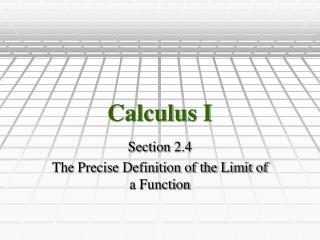# Calculus I - PowerPoint PPT PresentationDownload PresentationCalculus I

Download Presentation## Calculus I

- - - - - - - - - - - - - - - - - - - - - - - - - - - E N D - - - - - - - - - - - - - - - - - - - - - - - - - - -
##### Presentation Transcript

1. Calculus I Section 2.4 The Precise Definition of the Limit of a Function

2. Definition • The limit of f(x) as x approaches a is given by: • This limit exists if we can make values of f(x) arbitrarily close to L by taking x to be sufficiently close to a from either side, but not equal to a.

3. Definition • Let f be a function defined on some open interval that contains the number a, except possibly the number a itself. Then we say that the limit of f(x) as xapproaches a is L, and we write:if for every number e > 0 there exists a number d > 0 such that

4. YOWZA! • Say what? • Keep in mind that this is the formal way of saying that if the limit exists, then you can give me any e and I will be able to find a distance d from a that will keep f(x) within an e of L.

5. Definition of One-sided Limits

6. Infinite Limits • Let f be a function defined on some open interval that contains the number a, except possibly at a itself. Then: